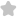based on alleu augi 0.5 If sin 0 = ...
Question# based on alleu augi 0.5 If sin 0 = and JEE/Engineering Exams Maths SolutionShare1094.0 (1 ratings)Walw of ( frac{sin theta+cos alpha}{tan theta} ) ( begin{aligned} frac{1}{r_{2}}+frac{1}{sqrt{2}} &=frac{2}{sqrt{2}} times frac{sqrt{2}}{sqrt{2}} &=frac{2}{2} sqrt{2} 0, &=sqrt{2} / 1 end{aligned} )1094.0 (1 ratings) Rate SolutionShareQuick and Stepwise Solutions Just click and SendOVER 20 LAKH QUESTIONS ANSWERED Download App for Free